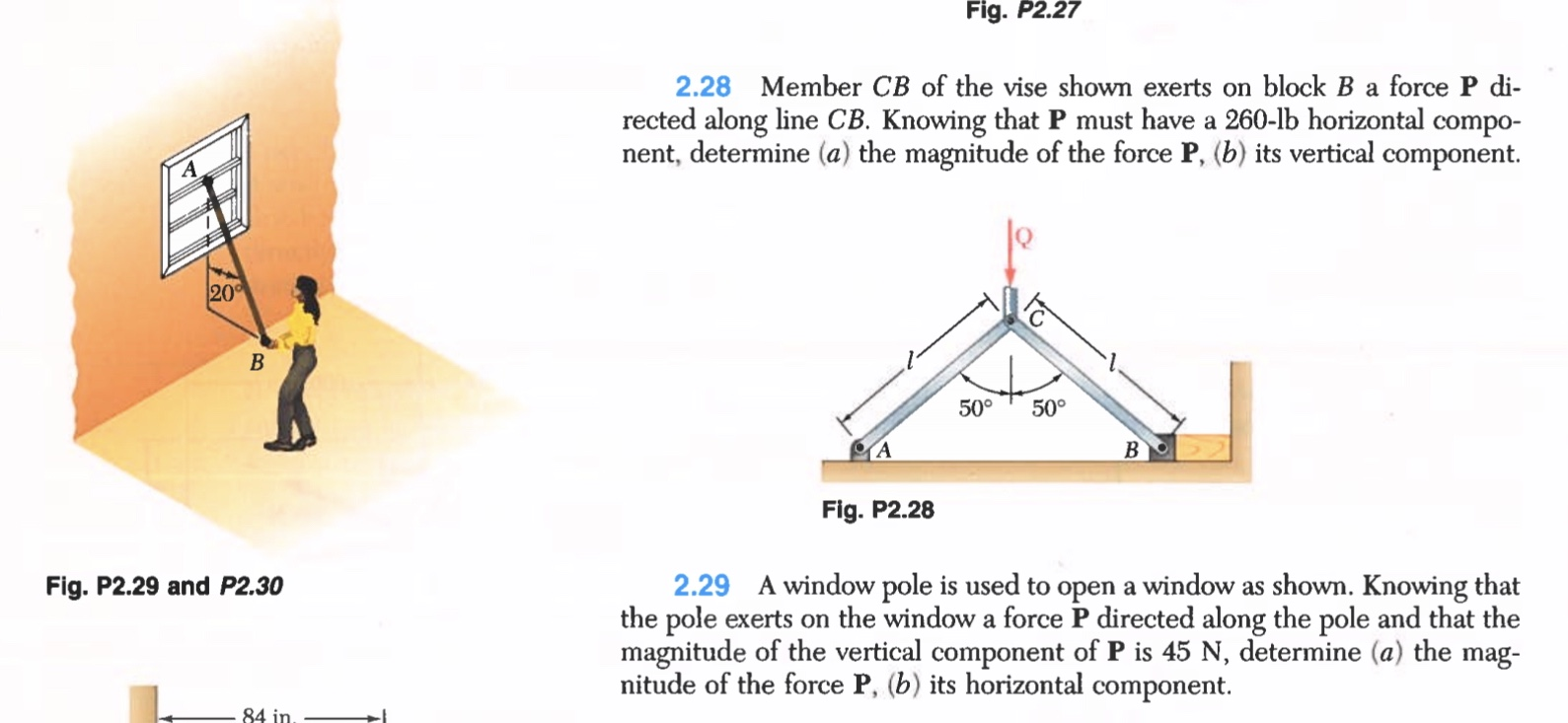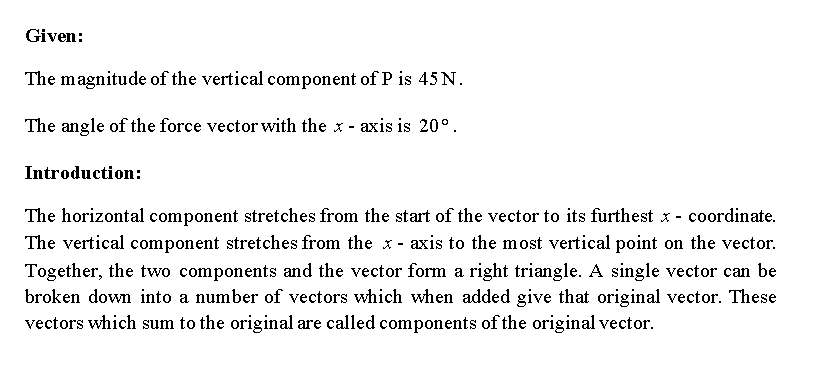# Member CB of the vise shown exerts on block B a force P di-rected along line CB. Knowing that P must have a 260-lb horizontal component, determine (a) the magnitude of the force P, (b) its vertical component.

Question-AnswerCategory: Engineering MechanicsMember CB of the vise shown exerts on block B a force P di-rected along line CB. Knowing that P must have a 260-lb horizontal component, determine (a) the magnitude of the force P, (b) its vertical component.

Member CB of the vise shown exerts on block B a force P di-rected along line CB. Knowing that P must have a 260-lb horizontal component, determine (a) the magnitude of the force P, (b) its vertical component.Step 1Step 2Step 3Step 4